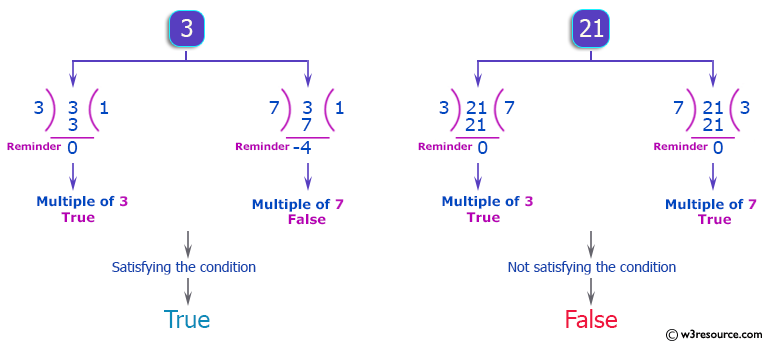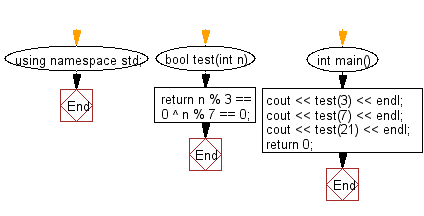﻿ C++ : If a number is a multiple of 3 or 7, but not both# C++ Exercises: Check if a given non-negative number is a multiple of 3 or 7, but not both

## C++ Basic Algorithm: Exercise-40 with Solution

Write a C++ program to check if a given non-negative number is a multiple of 3 or 7, but not both.

Sample Solution:

C++ Code :

``````#include <iostream>

using namespace std;

bool test(int n)
{
return n % 3 == 0 ^ n % 7 == 0;
}

int main()
{
cout << test(3) << endl;
cout << test(7) << endl;
cout << test(21) << endl;
return 0;
}
``````

Sample Output:

```1
1
0
```

Pictorial Presentation:Flowchart:C++ Code Editor: